Courses

# Previous Year Questions (2014-19) - The Solid State Notes | EduRev

## Class 12 : Previous Year Questions (2014-19) - The Solid State Notes | EduRev

The document Previous Year Questions (2014-19) - The Solid State Notes | EduRev is a part of the Class 12 Course Chemistry Class 12.
All you need of Class 12 at this link: Class 12

Q.1. An element has a body centered cubic (bcc) structure with a cell edge of 288 pm. The atomic radius is:    (2020)
A: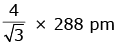B: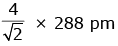C: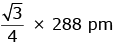D: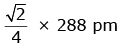Ans: C
For bcc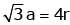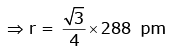Q.2. A compound is formed by cation C and anion A. The anions form hexagonal close packed (hcp) lattice and the cations occupy 75% of octahedral voids. The formula of the compound is :    (2019)
A: C2A3
B: C3A2
C: C3A4
D: C4A3
Ans:
C
Anions(A) are in hcp, so number of anions (A) = 6
Cations(C) are in 75% O.V., so number of cations (C)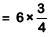= 18/4
= 9/2
So formula of compound will be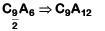C9A12 ⇒ C3A4

Q.3. Iron exhibits bcc structure at room temperature. Above 900°C, it transforms to fcc structure. The ratio of density of iron at room temperature to that at 900°C (assuming molar mass and atomic radii of iron remains constant with temperature) is    (2018)
A: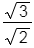B: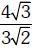C: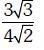D: 1/2
Ans:
C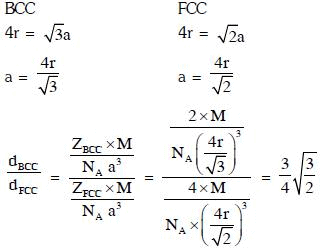Q.4. Which is the incorrect statement ?    (2017)
A: Density decreases in case of crystals with Schottky's defect
B: NaCl(s) is insulator, silicon is semi conductor,silver is conductor, quartz is piezo electric crystal
C: Frenkel defect is favoured in those ionic compounds in which sizes of cation and an ions are almost equal
D: FeO0.98 has non stoichiometric metal deficiency defect
Ans:
C
In frenkel defect the radius of cation must be very less than anion.

Q.5. Lithium has bcc structure. Its density is 530 kg m-3 and its atomic mass is 6.94 g mol-1. Calculate the edge length of a unit cell of Lithium metal. (NA = 6.02 x 1023 mol-1)    (2016)
A: 264 pm
B: 154 pm
C: 352 pm
D: 527 pm
Ans:
C
Given, Li has a bcc structure
Density (ρ) =530 kg-m-3
Atomic mass (M) = 6.94 g mol-1
Avogadro's number of atoms per unit cell in bcc (Z) = 2.
∴ We have the formula for density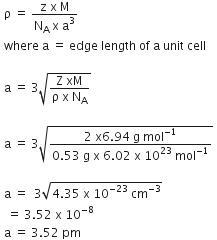Q.6. The ionic radii of A+ and B- ions are 0.98 x 10-10 m and 1.81 x10-10 m. The coordination number of each ions in AB is      (2016)
A: 2
B: 6
C: 4
D: 8
Ans:
B
Given, ionic radius of cation (A+) =0.98 x 10-10 m
Ionic radius of anion (B-)=1.81 x 10-10 m

Now we have,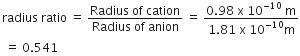If radius ratio range is in between 0.441-0.732, ion would have an octahedral structure. Therefore, the coordination number of each ion in AB is 6.

Q.7. A given metal crystallizes out with a cubic structure having edge length of 361 pm. If there are four metal atoms in one unit cell, what is the radius of one atom?    (2015)
A: 108 pm
B: 40 pm
C: 127 pm
D: 80 pm
Ans:
C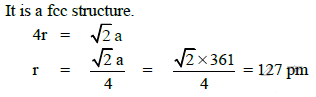Q.8. If 'a' is the length of the side of a cube, the distance between the body centered atom and one corner atom in the cube will be :    (2014)
A: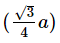B: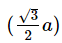C: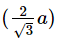D: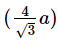Ans:
B
The distance between the body centered atom and corner atom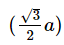Offer running on EduRev: Apply code STAYHOME200 to get INR 200 off on our premium plan EduRev Infinity!

## Chemistry Class 12

121 videos|250 docs|199 tests

,

,

,

,

,

,

,

,

,

,

,

,

,

,

,

,

,

,

,

,

,

;